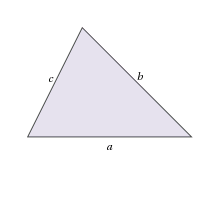PSAT Math : How to find the perimeter of an acute / obtuse triangle

Example Questions

Example Question #111 : Triangles

If a = 7 and b = 4, which of the following could be the perimeter of the triangle?I. 11

II. 15

III. 25

I and II Only

I Only

II and III Only

II Only

I, II and III

II Only

Explanation:

Consider the perimeter of a triangle:

P = a + b + c

Since we know a and b, we can find c.

In I:

11 = 7 + 4 + c

11 = 11 + c

c = 0

Note that if c = 0, the shape is no longer a trial. Thus, we can eliminate I.

In II:

15 = 7 + 4 + c

15 = 11 + c

c = 4.

This is plausible given that the other sides are 7 and 4.

In III:

25 = 7 + 4 + c

25 = 11 + c

c = 14.

It is not possible for one side of a triangle to be greater than the sum of both of the other sides, so eliminate III.

Thus we are left with only II.